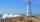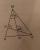High wall

I have a wall 2m high. I need a 15 degree angle (upward) to second wall 4 meters away. How high must the second wall?

Result

x =  3.07 m

Solution:Leave us a comment of example and its solution (i.e. if it is still somewhat unclear...):Be the first to comment!Next similar examples:

1. Internal anglesFind the internal angles of the triangle ABC if the angle at the vertex C is twice the angle at the B and the angle at the vertex B is 4 degrees smaller than the angle at the vertex A.
2. TreeHow tall is the tree that observed in the visual angle of 52°? If I stand 5 m from the tree and eyes are two meters above the ground.
3. FlowerbedFlowerbed has the shape of an isosceles obtuse triangle. Arm has a size 5.5 meters and an angle opposite to the base size is 94°. What is the distance from the base to opposite vertex?
4. ReflectorCircular reflector throws light cone with a vertex angle 49° and is on 33 m height tower. The axis of the light beam has with the axis of the tower angle 30°. What is the maximum length of the illuminated horizontal plane?
5. MapleMaple peak is visible from a distance 3 m from the trunk from a height of 1.8 m at angle 62°. Determine the height of the maple.
6. Reference angleFind the reference angle of each angle:
7. How farFrom the top of a lighthouse 145 ft above sea level, the angle of depression of a boat 29°. How far is the boat from the lighthouse?
8. Cable car 2Cable car rises at an angle 41° and connects the upper and lower station with an altitude difference of 1175 m. How long is the track of cable car?
9. LiftThe largest angle at which the lift rises is 16°31'. Give climb angle in permille.
10. CosineThe point (8, 6) is on the terminal side of angle θ. cos θ = ?
11. Triangle 42Triangle BCA. Angles A=119° B=(3y+14) C=4y. What is measure of triangle BCA=?
12. Angles in triangleThe triangle is ratio of the angles β:γ = 6:8. Angle α is 40° greater than β. What are the size of angles of the triangle?
13. Theorem proveWe want to prove the sentence: If the natural number n is divisible by six, then n is divisible by three. From what assumption we started?
14. ClimbFor horizontal distance 4.2 km road rise by 6.3 m. Calculate the road pitch in ‰ (permille, parts per thousand).
15. Obtuse angleThe line OH is the height of the triangle DOM, line MN is the bisector of angle DMO. obtuse angle between the lines MN and OH is four times larger than the angle DMN. What size is the angle DMO? (see attached image)
16. 30-60-90The longer leg of a 30°-60°-90° triangle measures 5. What is the length of the shorter leg?
17. Center traverseIt is true that the middle traverse bisects the triangle?